二维圆盘中不同外场对等离激元激发的影响Effect of the Different External Fields on Plasmon Excitations in Two-Dimensional Disk

• 全文下载: PDF(849KB)    PP.413-420   DOI: 10.12677/APP.2018.89052
• 下载量: 210  浏览量: 302   科研立项经费支持

We study the plasmon excitations in two-dimensional disk, based on the linear response theory in the random-phase approximation and the free-electron gas model. With the help of energy absorption spectrum and charge distribution, the effect of external electric fields on plasmon is obtained. Results show that the uniform external electric field only can excite quadrupole plasmon; the non-uniform external electric field can excite both quadrupole plasmon and quadrupole plasmon.

1. 引言

2. 研究方法

${\rho }^{ind}\left(r,\omega \right)=\int \Pi \left(r,{r}^{\prime },\omega \right)V\left({r}^{\prime },\omega \right)\text{d}{r}^{\prime }$ (1)

$\int \Pi \left(r,{r}^{\prime },\omega \right)=2{e}^{2}\underset{nm}{\sum }\frac{f\left({E}_{n}\right)-f\left({E}_{m}\right)}{{E}_{n}-{E}_{m}-\omega -i\gamma }{\psi }_{n}^{\ast }\left(r\right){\psi }_{m}\left(r\right){\psi }_{m}^{\ast }\left({r}^{\prime }\right){\psi }_{n}\left({r}^{\prime }\right)$ (2)

${V}^{ind}\left(r,\omega \right)=\frac{1}{4\text{π}{\epsilon }_{0}}\int \frac{{\rho }^{ind}\left({r}^{\prime },\omega \right)}{|r-{r}^{\prime }|}\text{d}{r}^{\prime }$ (3)

${V}^{ind}\left(r,\omega \right)=2{e}^{2}\underset{nm}{\sum }\frac{f\left({E}_{n}\right)-f\left({E}_{m}\right)}{{E}_{n}-{E}_{m}-\omega -i\gamma }×\frac{1}{4\text{π}{\epsilon }_{0}}\int \frac{{\psi }_{n}^{\ast }\left({r}^{\prime }\right){\psi }_{m}\left({r}^{\prime }\right)}{|r-{r}^{\prime }|}\text{d}{r}^{\prime }\left[{V}_{nm}^{ext}\left(\omega \right)+{V}_{nm}^{ind}\left(\omega \right)\right]$ (4)

${V}_{nm}\left(\omega \right)=\int {\psi }_{n}^{\ast }\left(r\right)V\left(r,\omega \right){\psi }_{m}\left(r\right)$ (5)

${V}_{{n}^{\prime }{m}^{\prime }}^{ind}\left(\omega \right)=2{e}^{2}\underset{nm}{\sum }\frac{f\left({E}_{n}\right)-f\left({E}_{m}\right)}{{E}_{n}-{E}_{m}-\omega -i\gamma }×\frac{1}{4\text{π}{\epsilon }_{0}}\int \frac{{\psi }_{n}^{\ast }\left({r}^{\prime }\right){\psi }_{m}\left({r}^{\prime }\right){\psi }_{{n}^{\prime }}^{\ast }\left(r\right){\psi }_{{m}^{\prime }}\left(r\right)}{|r-{r}^{\prime }|}\left[{V}_{nm}^{ext}\left(\omega \right)+{V}_{nm}^{ind}\left(\omega \right)\right]\text{d}{r}^{\prime }\text{d}r$ (6)

${M}_{{n}^{\prime }{m}^{\prime },nm}\left(\omega \right)=\frac{2{e}^{2}}{4\text{π}{\epsilon }_{0}}\underset{nm}{\sum }\frac{f\left({E}_{n}\right)-f\left({E}_{m}\right)}{{E}_{n}-{E}_{m}-\omega -i\gamma }\int \frac{{\psi }_{{n}^{\prime }}^{\ast }\left(r\right){\psi }_{{m}^{\prime }}\left(r\right){\psi }_{n}^{\ast }\left({r}^{\prime }\right){\psi }_{m}\left({r}^{\prime }\right)}{|r-{r}^{\prime }|}\text{d}{r}^{\prime }\text{d}r$ (7)

$\underset{nm}{\sum }\left[{\delta }_{{n}^{\prime }{m}^{\prime },nm}-{M}_{{n}^{\prime }{m}^{\prime },nm}\left(\omega \right)\right]{V}_{nm}^{ind}\left(\omega \right)=\underset{nm}{\sum }{M}_{{n}^{\prime }{m}^{\prime },nm}\left(\omega \right){V}_{nm}^{ext}\left(\omega \right)$ (8)

$A\left(\omega \right)=-\frac{1}{2}\omega \mathrm{Im}\left\{\int {\rho }^{ind}\left(r,\omega \right){\left[{V}^{ext}\left(r,\omega \right)\right]}^{\ast }\right\}$ (9)

${\psi }_{nk}\left(r,\theta \right)=\sqrt{\frac{1}{\text{π}{R}_{\text{0}}^{\text{2}}}}\frac{{J}_{n}\left(\frac{{x}_{nk}}{{R}_{\text{0}}^{\text{2}}}r\right)}{{J}_{n-1}\left({x}_{nk}\right)}{e}^{±in\theta }$ (10)

${E}_{nk}=\frac{{\hslash }^{2}{x}_{nk}^{2}}{2{m}_{e}{R}_{\text{0}}^{\text{2}}}$ (11)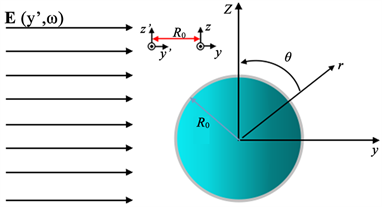Figure 1. The two-dimensional disk model in the external electric field incident

${\psi }_{nk}\left(r,\theta \right)=C{R}_{n}\left({N}_{k}r\right){e}^{±in\theta }$ (12)

${\rho }^{ind}\left(r,\theta ,\omega \right)=\underset{m}{\sum }{\rho }_{m}^{ind}\left(r,\omega \right){e}^{im\theta }$ (13)

${V}^{ind}\left(r,\theta ,\omega \right)=\underset{m}{\sum }{V}_{m}^{ind}\left(r,\omega \right){e}^{im\theta }$ (14)

$\begin{array}{c}{\rho }_{m}^{ind}\left(r,\omega \right)=4\text{π}{e}^{2}\left[\underset{nk,{k}^{\prime }}{\sum }\left(\frac{1}{{E}_{nk}-{E}_{\left(n+m\right){k}^{\prime }}-\omega -i\gamma }+\frac{1}{{E}_{nk}-{E}_{\left(n+m\right){k}^{\prime }}+\omega +i\gamma }\right)\right]\\ ×{C}^{2}{R}_{n}\left({N}_{k}r\right){R}_{n+m}\left({N}_{{k}^{\prime }}r\right)\left[{V}_{nk{k}^{\prime }}^{ext}\left(\omega \right)+{V}_{nk{k}^{\prime }}^{ind}\left(\omega \right)\right]\end{array}$ (15)

${V}_{m}^{ind}\left(r,\omega \right)=\int \frac{{r}^{\prime }{\rho }_{m}^{ind}\left({r}^{\prime },\omega \right){\text{e}}^{im\left(\theta -{\theta }^{\prime }\right)}}{4\text{π}{\epsilon }_{0}\sqrt{{r}^{2}+{{r}^{\prime }}^{2}-2r{r}^{\prime }\mathrm{cos}\left(\theta -{\theta }^{\prime }\right)}}\text{d}{r}^{\prime }\text{d}\left(\theta -{\theta }^{\prime }\right)$ (16)

${V}_{nk{k}^{\prime }}\left(\omega \right)={C}^{2}\int {r}^{\prime }{R}_{n+m}\left({N}_{{k}^{\prime }}{r}^{\prime }\right){R}_{n}\left({N}_{k}{r}^{\prime }\right){V}_{m}\left({r}^{\prime },\omega \right)\text{d}{r}^{\prime }$ (17)

$\underset{nk{k}^{\prime }}{\sum }\left[{\delta }_{ab{b}^{\prime }}-{M}_{ab{b}^{\prime },nk{k}^{\prime }}\left(\omega \right)\right]{V}_{nk{k}^{\prime }}^{ind}\left(\omega \right)=\underset{nk{k}^{\prime }}{\sum }{M}_{ab{b}^{\prime },nk{k}^{\prime }}\left(\omega \right){V}_{nk{k}^{\prime }}^{ext}\left(\omega \right)$ (18)(19)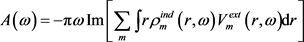(20)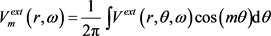(21)

3. 结果与分析Figure 2. Energy Absorption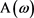with frequencyin the uniform external electric field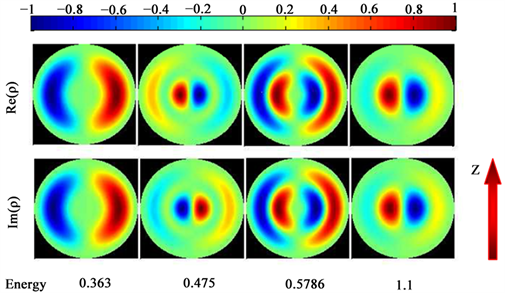Figure 3. The charge distribution of the plasmon excited by the uniform electric field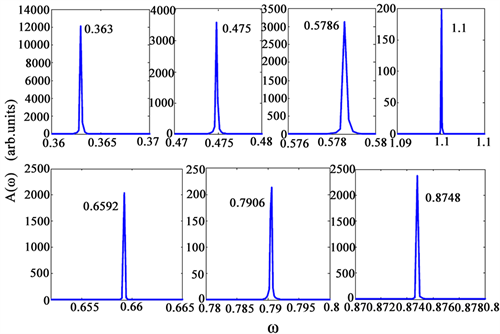Figure 4. Energy absorptionwith frequencyin the non-uniform electric field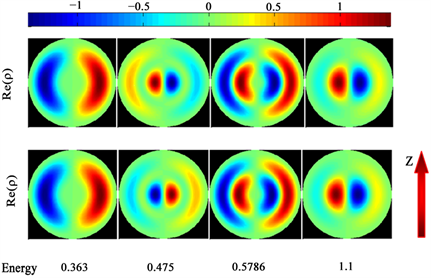Figure 5. The charge distribution of the plasmon excited by both the uniform field and the non-uniform field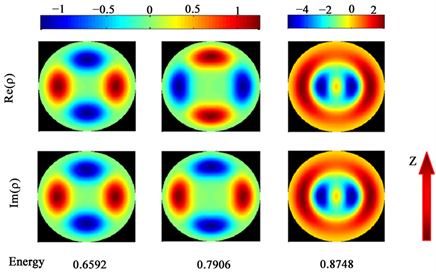Figure 6. The charge distribution of the plasmon excited only by the non-uniform field

4. 结论

NOTES

*通讯作者。

  Huang, H., Wang, B. and Long, H. (2014) Plasmon-Negative Refraction at the Heterointerface of Graphene Sheet Arrays. Optics Letters, 39, 5957-5960. https://doi.org/10.1364/OL.39.005957  龚健, 张利伟, 陈亮. 石墨烯基双曲色散特异材料的负折射与体等离子体性[J]. 物理学报, 2015, 64(6): 67301-067301.  Ghenuche, P., Cherukulappurath, S. and Taminiau, T.H (2008) Spectroscopic Mode Mapping of Resonant Plasmon Nanoantennas. Physical Review Letters, 101, 116805-116808. https://doi.org/10.1103/PhysRevLett.101.116805  Kim, S., Jin, J. and Kim, Y. (2008) High-Harmonic Generation by Reso-nant Plasmon Field Enhancement. Nature, 453, 757-760. https://doi.org/10.1038/nature07012  Mühlschlegel, P., Eisler, H.J. and Martin, O.J.F. (2005) Resonant Optical Antennas. Science, 308, 1607-1609. https://doi.org/10.1126/science.1111886  尹海峰, 毛力. 一维原子链局域等离激元的非线性激发[J]. 物理学报, 2016, 65(8): 321-327.  Auguié, B. and Barnes, W.L. (2008) Collective Resonances in Gold Nanoparticle Arrays. Physical Review Letters, 101, 143902-143905. https://doi.org/10.1103/PhysRevLett.101.143902  Hicks, E.M., Zou, S. and Schatz, G.C. (2005) Controlling Plasmon Line Shapes through Diffractive Coupling in Linear Arrays of Cylindrical Nanoparticles Fabricated by Electron Beam Lithography. Nano Letters, 5, 1065-1070. https://doi.org/10.1021/nl0505492  Chu, Y., Schonbrun, E. and Yang, T. (2008) Experimental Observation of Narrow Sur-face Plasmon Resonances in Gold Nanoparticle Arrays. Applied Physics Letters, 93, 181108-181110. https://doi.org/10.1063/1.3012365  Sun, Z., Jung, Y.S. and Kim, H.K. (2003) Role of Surface Plasmons in the Optical In-teraction in Metallic Gratings with Narrow Slits. Applied Physics Letters, 83, 3021-3023. https://doi.org/10.1063/1.1618021  Barnes, W.L., Murray, W.A. and Dintinger, J. (2004) Surface Plasmon Polaritons and Their Role in the Enhanced Transmission of Light through Periodic Arrays of Subwavelength Holes in a Metal Film. Physical Review Letters, 92, 107401-107404. https://doi.org/10.1103/PhysRevLett.92.107401  Wang, Q.J., Huang, C.P. and Li, J.Q. (2006) Suppression of Transmission Minima and Maxima with Structured Metal Surface. Applied Physics Letters, 89, 221121-221123. https://doi.org/10.1063/1.2400098  Fang, X., Li, Z. and Long, Y. (2007) Surface-Plasmon-Polariton Assisted Diffraction in Periodic Subwavelength Holes of Metal Films with Reduced Interplane Coupling. Physical Review Letters, 99, 066805-066808. https://doi.org/10.1103/PhysRevLett.99.066805  Xue, H.J., Hao, D.P, Zhang, M. and Wang, X.M. (2017) Plasmon Excita-tions in the Dimers Formed by Atom Chains. Physica E, 86, 292-296. https://doi.org/10.1016/j.physe.2016.10.030  Xue, H.J., Wu, R.L. and Hu, C.X. (2018) The Study of the Plasmon Modes of Square Atomic Clusters Based on the Eigen-Oscillation Equation of Charge under the Free-Electron Gas Model. International Journal of Modern Physics B, 32, 1850139-1850148. https://doi.org/10.1142/S0217979218501394